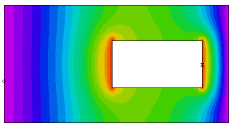﻿ Sample Problems > Usage > 3D_domains > 3d_void

# 3d_void

Navigation:  Sample Problems > Usage > 3D_domains >

# 3d_void{ 3D_VOID.PDE

This example shows the use of empty layers in 3D applications.

The VOID statement appears inside a REGION section, in the position of a

layer parameter definition.

The syntax is:

LAYER number VOID

This statement causes the stated layer to be excluded from the problem domain

in the current REGION. (Remember that a REGION refers to a partition of the

2D projection plane.)

Boundary conditions on the surface of the void are specified by the standard

boundary condition facilities.

In this problem, we have a heat equation with an off-center void in an irregular

figure.  The Y faces held at zero, the Z-faces are insulated, and the sides

of the void are held at 1.

}

 title '3D VOID LAYER TEST'   coordinates    cartesian3   select    errlim = 0.005   variables    u   definitions    k = 0.1    h=0    x0=0.2  y0=-0.3    x1=1  y1 = 0.3   equations    U: div(K*grad(u)) + h = 0   extrusion z=0, 0.3, 0.7, 1boundaries

region 1

start(-1,-1)

value(u)=0           { Force U=0 on perimeter }

line to (1,-1)

arc(center=-1,0) to (1,1)

line to (-1,1)

arc(center= -3,0) to close

limited region 2       { void exists only on layer 2 }

layer 2 VOID

start(x0,y0)

layer 2 value(u)=1

line to (x1,y0) to (x1,y1) to (x0,y1) to close

monitors

elevation(u) from (-0.8,0,0.5) to (1.25,0,0.5)

elevation(u) from (-0.8,0,0.8) to (1.25,0,0.8)

contour(u) on z=0

contour(u) on z=0.5

contour(u) on z=1

contour(u) on y=0

plots

elevation(u) from (-0.8,0,0.5) to (1.25,0,0.5)

elevation(u) from (-0.8,0,0.8) to (1.25,0,0.8)

contour(u) on z=0       painted

contour(u) on z=0.5     painted

contour(u) on z=0.499   painted

contour(u) on z=1       painted

contour(u) on y=0       painted

end